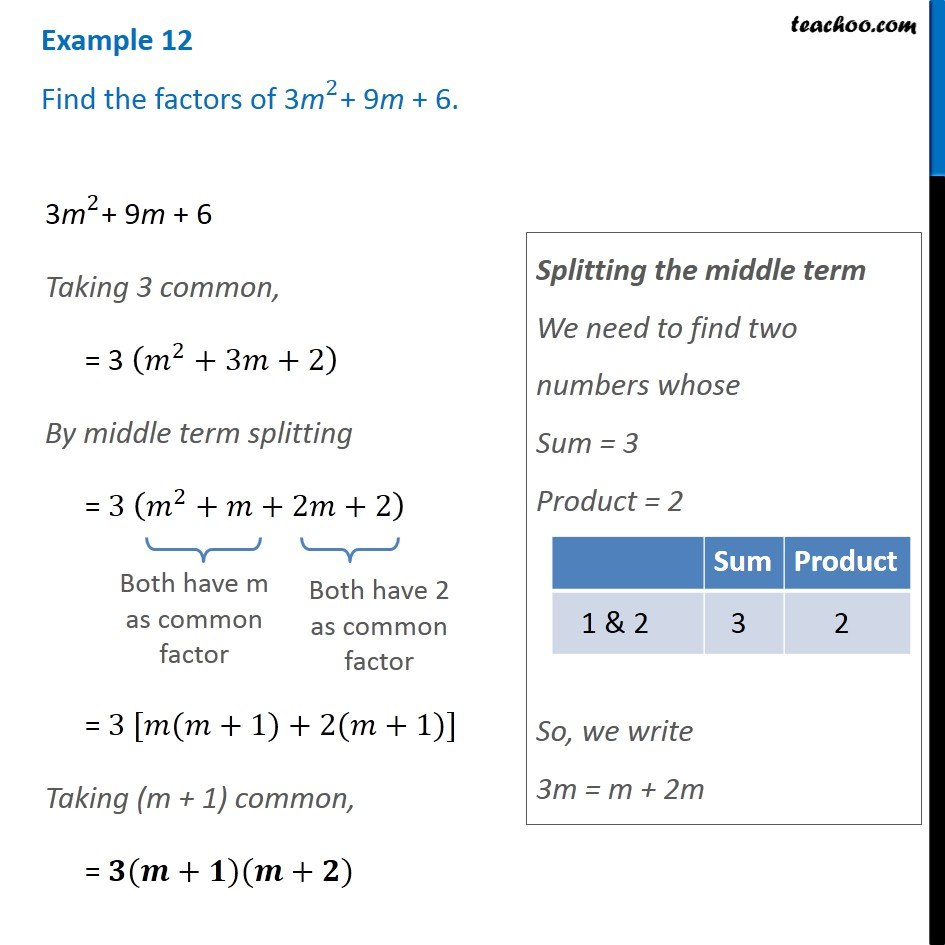Factorisation using splitting middle term

Chapter 14 Class 8 Factorisation
Concept wiseIntroducing your new favourite teacher - Teachoo Black, at only ₹83 per month

### Transcript

Example 12 Find the factors of 〖"3m" 〗^2+ 9m + 6. 〖"3m" 〗^2+ 9m + 6 Taking 3 common, = 3 (𝑚^2+3𝑚+2) By middle term splitting = 3 (𝑚^2+𝑚+2𝑚+2) Both have m as common factor Both have 2 as common factor = 3 [𝑚(𝑚+1)+2(𝑚+1)] Taking (m + 1) common, = 𝟑(𝒎+𝟏)(𝒎+𝟐) Splitting the middle term We need to find two numbers whose Sum = 3 Product = 2 So, we write 3m = m + 2m